# The 100th Day of School

Kindergarten and first-grade students from coast to coast celebrate the 100th Day of school. They read books about 100 and the 100th day of school. They bring collections of 100 items to school, and they also complete many 100th day worksheets.

My blogging friend, Paula Krieg, designed a fabulous art project for second-grade students. I just had to update this post and share her work with you. Her project would also be perfect for the 100th day of school for the mid-elementary grades. In the video near the end of her post, she explains her 100¢ art project. Her post includes detailed instructions, templates of everything needed to complete the project, and links showing completed projects. Check it out!

I like the Hap Palmer song about coins. It happens to teach important multiplication facts, including some about the number 100. Third graders could learn these multiplication facts on the 100th day of school. This song would also go very well with Paula’s 100¢ project above:

You will also love the games, puzzles, art projects, etc. suggested in MathGeekMama’s 100 Math Ideas for the 100th Day of School.

What about students in 4th or 5th grade or even middle school or high school? Can older students celebrate the 100th day of school? I think so! Share some of the following information with them:

Here is the basic factoring information for the number 100.

• 100 is a composite number.
• Prime factorization: 100 = 2 × 2 × 5 × 5, which can be written 100 = 2²× 5²
• The exponents in the prime factorization are 2 and 2. Adding one to each and multiplying we get (2 + 1)(2 + 1) = 3 × 3 = 9. Therefore 100 has exactly 9 factors.
• Factors of 100: 1, 2, 4, 5, 10, 20, 25, 50, 100
• Factor pairs: 100 = 1 x 100, 2 x 50, 4 x 25, 5 x 20, or 10 x 10
• 100 is a perfect square. √100 = 10Here are a few of several possible factor trees that can be made for the number 100: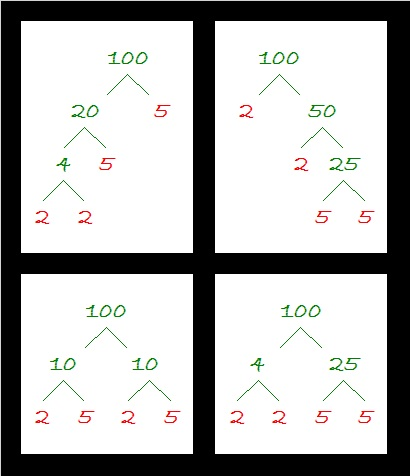What about the factors of all the numbers from 1 to 100? Here’s a clever chart giving those factors. Click on the tweet to see the whole chart.

What do you think the area of the following shape will be?You got it, 100 cm².

Since √100 = 10, a whole number, 100 is a perfect square. Did you know that 100 can be written as the sum of TWO OTHER perfect squares?

• 36 + 64 = 100. In other words, 6² + 8² = 10²

36, 64, and 100 were all included as clues in the very first puzzle I published on this blog:Note: When 100 is a clue in a FIND THE FACTORS puzzles, always use 10 × 10. Even though 100 has other factors, 10 × 10 is its only factor pair in which both factors are ten or less.

100 is the sum of a few different types of consecutive numbers:

• 100 = 18 + 19 + 20 + 21 + 22; that’s the sum of 5 consecutive numbers
• 100 = 9 + 10 + 11 + 12 + 13 + 14 + 15 + 16; that’s the sum of 8 consecutive numbers
• 100 = 22 + 24 + 26 + 28; that’s the sum of 4 consecutive EVEN numbers
• 16 + 18 + 20 + 22 + 24 = 100; that’s the sum of 5 consecutive EVEN numbers
• 1 + 3 + 5 + 7 + 9 + 11 + 13 + 15 + 17 + 19 = 100; that’s the sum of the first 10 ODD numbers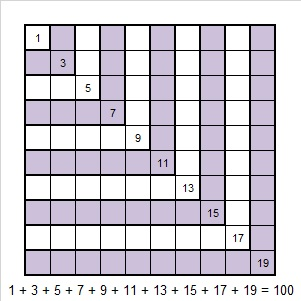• (1 + 2 + 3 + 4)² = 100; that the square of the sum of the first four numbers
• 100 = 2 + 3 + 5 + 7 + 11 + 13 + 17 + 19 + 23; that’s the sum of the first 9 prime numbers.
• 100 = 47 + 53; that’s the sum of two consecutive prime numbers
• 1³ + 2³ + 3³ + 4³ = 100, that’s the sum of the first four cubes

100 is the hypotenuse of two Pythagorean triple triangles:

• 60² + 80² = 100²
• 28² + 96² = 100²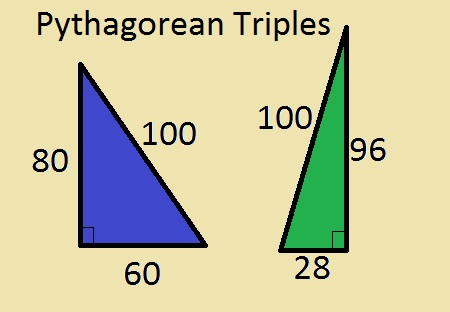But 100 is a leg in SEVERAL other Pythagorean triples:

• 75² + 100² = 125²
• 100² + 105² = 145²
• 100² + 240² = 260²
• 100² + 495² = 505²
• 100² + 621² = 629²
• 100² + 1248² = 1252²
• 100² + 2499² = 2501²

That last one is called a Primitive Pythagorean triple because the greatest common factor of 100, 2499, and 2501 is one. Can you find the greatest common factor of any of the other triples?

Here’s the ONE non-trivial way 100 is the difference of two squares:

• 26² – 24² = 100, and that’s because (26 + 24)(26 – 24) = 100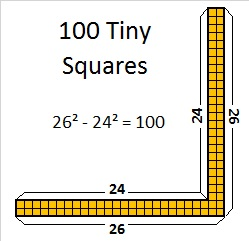100 is a palindrome in five different bases (Palindromes read the same backward or forwards such as “Madam, I’m Adam” or “race car”):

• 10201 in BASE 3 because 1(3⁴) + 0(3³) + 2(3²) + 0(3¹) + 1(3º) = 100
• 202 in BASE 7 because 2(7²) + 0(7¹) + 2(7º) = 100
• 121 BASE 9 because 1(9²) + 2(9¹) + 1(9º) = 100
• 55 BASE 19 because 5(19¹) + 5(19º) = 100
• 44 BASE 24 because 4(24¹) + 4(24º) = 100

If you convert 100 from base 10 to these other bases, you will get:

• 40 in BASE 25
• 50 in BASE 20
• 400 in BASE 5

We can find numbers that have 100 factors by using prime factorizations. This chart lists some candidates for the smallest number with 100 factors. Notice that to make each option as small as possible, the largest exponent goes with the smallest prime number in each prime factorization. Can you order these nine numbers from smallest to greatest?The smallest number to have exactly 100 factors is 45,360. Can you list all 100 of those factors?

Now for today’s puzzle. . .100 isn’t one of its clues, but can you find the one and only place where 100 belongs in this mixed up multiplication table?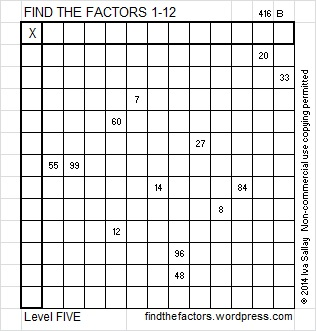This week’s puzzles and last week’s solutions: 12 Factors 2014-04-21

I hope you fully enjoy the 100th day of school!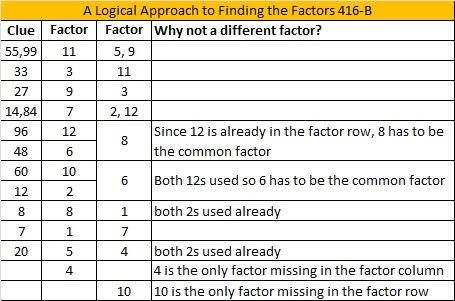This site uses Akismet to reduce spam. Learn how your comment data is processed.